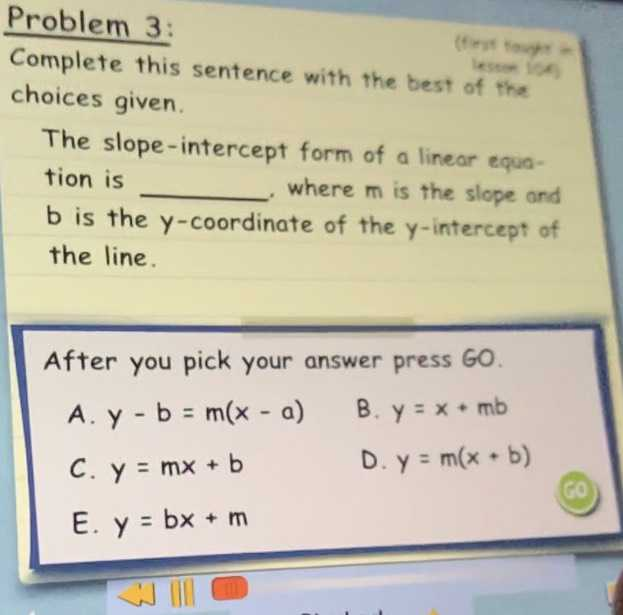### ¿Todavía tienes preguntas de matemáticas?

Pregunte a nuestros tutores expertos
Algebra
PreguntaComplete this sentence with the best of the choices given. The slope-intercept form of a linear equa- tion is

$$b$$ is the $$y$$ -coordinate of the $$y$$ -intercept of the line. A. $$y - b = m ( x - a )$$ B. $$y = x + m b$$

C. $$y = m x + b$$ D. $$y = m ( x + b )$$

E. $$y = b x + m$$